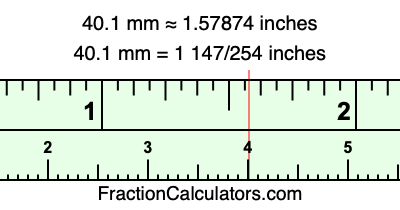40.1 mm in inchesHere is how to convert 40.1 mm to inches. We will give you the fractional answer, the decimal answer, and illustrate the answer on our tape measure.

One millimeter is equal to 5/127 inches, and 40.1 as a fraction is 401/10. Therefore, to get the fractional answer to 40.1 mm in inches, we multiply 401/10 by 5/127 and simplify if necessary.

cm × 5/127 = inches
401/10 × 5/127 = inches
401/10 × 5/127 = 1 147/254
401/10 mm = 1 147/254 inches
40.1 mm = 1 147/254 inches

As promised above, we also have the answer to 40.1 mm in inches in decimal form. Although the fractional answer above is exact, the decimal answer may be rounded if necessary:

1 147/254 ≈ 1.57874015748031
1 147/254 ≈ 1.57874
40.1 mm ≈ 1.57874 inches

Our image below shows 40.1 mm on a tape measure. The top row of the tape measure is inches, and each mark on the bottom row is 1 millimeter (10 millimeter marks equal 1 centimeter).As you can see, we drew a red line where 40.1 mm and 1.57874 inches meet on the measuring tape.

Millimeters to Inches Calculator
Here you can convert another length in millimeters to inches.

Convert  mm to inches.

What is 40.2 mm in inches?
Here is another inches to millimeters calculation that we have done for you.